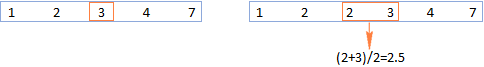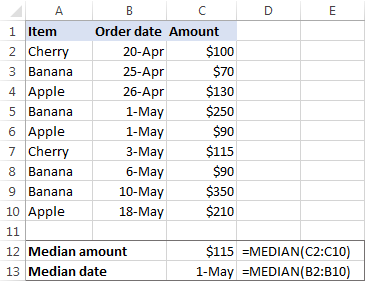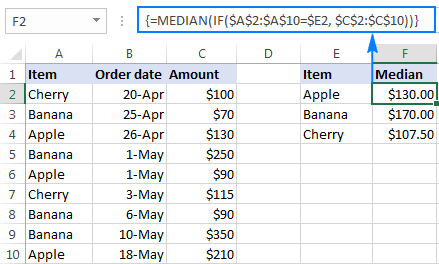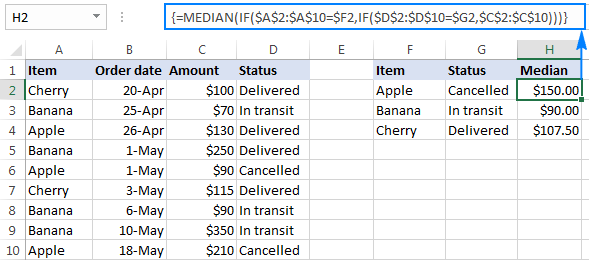# How to calculate median in Excel - formula examples

The tutorial shows how to use the MEDIAN function to calculate a median of numeric values in Excel.

The median is one of the three main measures of central tendency, which is commonly used in statistics for finding the center of a data sample or population, e.g. for calculating a typical salary, household income, home price, real-estate tax, etc. In this tutorial, you will learn the general concept of the median, in what way it is different from arithmetic mean, and how to calculate it in Excel.

## What is median?

In simple terms, the median is the middle value in a group of numbers, separating the higher half of values from the lower half. More technically, it is the center element of the data set arranged in order of magnitude.

In a data set with an odd number of values, the median is the middle element. If there are an even number of values, the median is the average of the middle two.

For example, in the group of values {1, 2, 3, 4, 7} the median is 3. In the dataset {1, 2, 2, 3, 4, 7} the median is 2.5.Compared to the arithmetic mean, the median is less susceptible to outliers (extremely high or low values) and therefore it is the preferred measures of central tendency for an asymmetrical distribution. A classic example is a median salary, which gives a better idea of how much people typically earn than an average salary because the latter may be skewed by a small number of abnormally high or low salaries. For more information, please see Mean vs. median: which is better?

## Excel MEDIAN function

Microsoft Excel provides a special function to find a median of numeric values. Its syntax is as follows:

MEDIAN(number1, [number2], …)

Where Number1, number2, … are numeric values for which you want to calculate the median. These can be numbers, dates, named ranges, arrays, or references to cells containing numbers. Number1 is required, subsequent numbers are optional.

In Excel 2007 and higher, the MEDIAN function accepts up to 255 arguments; in Excel 2003 and earlier you can only supply up to 30 arguments.

### 4 facts you should know about Excel Median

• When the total number of values is odd, the function returns the middle number in the data set. When the total number of values is even, it returns an average of the two middle numbers.
• Cells with zero values (0) are included in calculations.
• Empty cells as well as cells containing text and logical values are ignored.
• The logical values TRUE and FALSE typed directly in formula are counted. For example, the formula MEDIAN(FALSE, TRUE, 2, 3, 4) returns 2, which is the median of the numbers {0, 1, 2, 3, 4}.

## How to calculate median in Excel - formula examples

MEDIAN is one of the most straightforward and easy-to-use functions in Excel. However, there are still some tricks, not obvious to beginners. Say, how do you calculate a median based on one or more conditions? The answer is in one of the following examples.

### Excel MEDIAN formula

For starters, let's see how to use the classic MEDIAN formula in Excel to find the middle value in a set of numbers. In a sample sales report (please see the screenshot below), supposing you want to find the median of numbers in cells C2:C8. The formula would be as simple as this:

`=MEDIAN(C2:C8)`As shown in the screenshot above, the formula works for numbers and dates equally well since in terms of Excel dates are also numbers.

### Excel MEDIAN IF formula with one criterion

Regrettably, Microsoft Excel does not provide any special function to calculate a median based on a condition like it does for the arithmetic mean (AVERAGEIF and AVERAGEIFS functions). Luckily, you can easily build your own MEDIAN IF formula in this way:

MEDIAN(IF(criteria_range=criteria, median_range))

In our sample table, to find a median amount for a specific item, input the item name in some cell, say E2, and use the following formula to get the median based on that condition:

`=MEDIAN(IF(\$A\$2:\$A\$10=\$E2, \$C\$2:\$C\$10))`

The formula tells Excel to calculate only those numbers in column C (Amount) for which a value in column A (Item) matches the value in cell E2.

Please pay attention that we use the \$ symbol to create absolute cell references. It is especially important if you intend to copy your Median If formula to other cells.

Finally, since you want to check every value in the specified range, make it an array formula by pressing Ctrl + Shift + Enter. If done correctly, Excel will encapsulate the formula in curly braces like shown in the screenshot below.

In dynamic array Excel (365 and 2021) it also works as a regular formula.### Excel Median IFS formula with multiple criteria

Taking the previous example further, let's add one more column (Status) to the table, and then find a median amount for each item, but count only orders with the specified status. In other words, we will be calculating median based on two conditions - item name and order status. To express multiple criteria, use two or more nested IF functions, like this:

MEDIAN(IF(criteria_range1=criteria1, IF(criteria_range2=criteria2, median_range)))

With criteria1 (Item) in cell F2 and criteria2 (Status) in cell G2, our formula takes the following shape:

`=MEDIAN(IF(\$A\$2:\$A\$10=\$F2, IF(\$D\$2:\$D\$10=\$G2,\$C\$2:\$C\$10)))`

Since it's an array formula, remember to hit Ctrl + Shift + Enter to complete it correctly. If all done properly, you will get a result similar to this:This is how you calculate median in Excel. To have a closer look at the formulas discussed in this tutorial, you are welcome to download our sample workbook below. I thank you for reading and hope to see you on our blog next week!

## Practice workbook

MEDIAN formula Excel - examples (.xlsx file)

## You may also be interested in

1. Using Excel from the Office 365 suite of applications, in Portuguese, a police officer needs to divide the sum of the range of cells from A3 to A6 by the average of the range of cells from B3 to B6. In the cell that will receive the result, you must use the formula:
A) =SUM (A3;A6) /MEAN (B3;B6)
B) =SUM (A3,A6) /MEAN(B3,B6)
C) =SUM (A3:A6) /MEAN (B3:B6)
D) =SUM (A3..A6) /MEAN (B3..B6)
E) =SUM (A3+A4+A5+A6) /MEAN (B3+B4+B5+B6)

is it correct to say that both the letter "c" and also the letter "d" get the same results?

• Check it out for yourself using your own data.

2. In expansion 0f mean, mode, median with forumula
following table with example as stastical average base results.

3. I have put the same furmula but it's not working

4. How would I go if I want to calculate a median only if there are 3 results or more, and have "n/a" for results in the cell if there are only 1 or 2 results? I need it in the same formula, to be able to copy and paste in multiple individual tables.
scenario 1 - need to calculate median
\$719.00
\$924.00
\$942.00
\$1,090.00
\$700.00
scenario 2 - need "n/a" as a result of the formula
\$1,090.00
\$700.00
Thank you!

5. I am using a an array to calculate the median {=MEDIAN(IF('2 Full Pay Relevant'!\$B\$2:\$B\$305='GPG Calca'!A19,'2 Full Pay Relevant'!\$AB\$2:\$AB\$305))} and cross-checking it with data values from a pivot table for the same data range. But this produces a different answer.
I've checked previous calculations that produced the same answer from both, but for the life of me can't see why. Do you have any suggestions for what I should look at, please?

6. I tried to calculate the median with multiple criteria using if AND condition instead of nested if,it is giving wrong answer.Why this is so? Since nested if and AND can be a repplacement of each other?Can you justify

• Jaiprakash:
Are the ranges in your statements absolute? Are the cells preceded by the "\$"?
These multiple IF/AND statements need to be an array. After you created the statement did you CTRL-Shift-Enter. That will create the curly brackets to surround the formula that tells Excel this should be seen as an array.

7. l;'

8. how to remove number if text and number mention in one column as like (JAGDISH IS WORKI,NG SINCE 3903702016 BUT THERE IS PROME WITH WORK
)

• Hello,

Please try to solve your task with the help of the Remove Characters tool which is a part of our Ultimate Suite for Excel. You can download its fully functional 14-day trial version using this direct link.
After you install the product, you will find Remove Characters in the Text section under the Ablebits Data tab.

9. I'm trying to calculate a median for a large data set.
Column b being ages (b5:b100)
Column c being number of people if that age. (c5:c100).